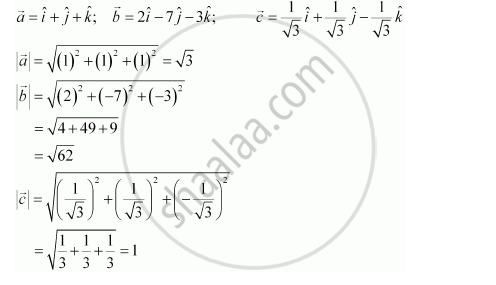Share

# Compute the Magnitude of the Following Vectors - CBSE (Science) Class 12 - Mathematics

ConceptMultiplication of a Vector by a Scalar

#### Question

Compute the magnitude of the following vectors:

veca = hati + hatj + hatk; vecb = 2hati - 7hatj - 3hatk;  vecc = 1/sqrt3 hati + 1/sqrt3 hatj - 1/sqrt3 hatk

#### Solution

The given vectors are:Is there an error in this question or solution?

#### Video TutorialsVIEW ALL 

Solution Compute the Magnitude of the Following Vectors Concept: Multiplication of a Vector by a Scalar.
S